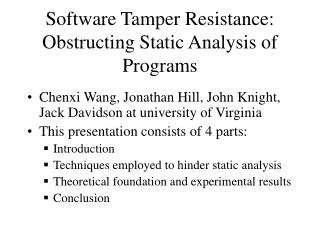DownloadDownload PresentationSoftware Tamper Resistance: Obstructing Static Analysis of Programs

# Software Tamper Resistance: Obstructing Static Analysis of Programs

Télécharger la présentation## Software Tamper Resistance: Obstructing Static Analysis of Programs

- - - - - - - - - - - - - - - - - - - - - - - - - - - E N D - - - - - - - - - - - - - - - - - - - - - - - - - - -
##### Presentation Transcript

1. Software Tamper Resistance: Obstructing Static Analysis of Programs • Chenxi Wang, Jonathan Hill, John Knight, Jack Davidson at university of Virginia • This presentation consists of 4 parts: • Introduction • Techniques employed to hinder static analysis • Theoretical foundation and experimental results • Conclusion

2. Introduction • Problem addressed: protecting trusted software against tampering on untrustworthy hosts. • The solution: tamper resistant software. Why is access control infeasible? • One aspect of software tamper resistance: prevention of static analysis of programs.

3. Static Analysis of Programs • “Static analysis refers to techniques designed to extract [semantic] information from a static image of a computer program.” • Entails two steps: control-flow analysis and data-flow analysis. • Can be defeated by making the program control-flow data-dependent.

4. Control-flow Transformations • Modify high-level control transfers to obstruct static detection of branch targets and call destinations. • Two steps of transformation are illustrated in the following figures.

5. int a,b;a=1;b=2;while(a<10){ b=a+b; if(b>10) b--; a++;}use(b); L1: if(!(a<10)) goto L4 Dismantling of High-level Constructs a=1 b=2 b=a+b if(!(b>10)) goto L2 b-- L2: a++ goto L1 L4: use (b)

6. Transform to indirect control transfers swVar=1 switch(swVar) L1: a=1; b=2; swVar=2; L2: if(!(a<10)) swVar=6; else swVar=3; L3: b=b+a; if(!(b>10)) swVar=5; else swVar=4; L4: b--; swVar=5; L5: a++; swVar=2; L6: use(b); goto switch

7. Data-flow Transformations • Dynamic computation of branch targets. • The introduction of non-trivial aliases into the program.

8. dynamic computation of the switch variable int global_array[]; switch(swVar) switch(swVar) L3: b=b+a; if(!(b>10)) swVar=5; else swVar=4; L3: b=b+a; if(!(b>10)) swVar=global_array[f1()]; else swVar=global_array[f2()]; goto switch; goto switch

9. Introducing aliases through pointers *p = a; a = a +b; *p = b b = 3; L1: *p = a; a = a+b; swVar = f1(); L2: *p = b; b = 3; swVar = f2(); A <*p,b> A <*p,a><*p,b>

10. Complexity Evaluation • Theorem 1: in the presence of general pointers, the problem of determining precise indirect branch target addresses is NP-hard. • Proof: constructing a polynomial time reduction from 3SAT problem to it. • Cannot be solved in polynomial time under the assumption that P!=NP.

11. Experimental Results • Transformations considerably hindered the optimization that the compiler(gcc) is able to perform. • Defeated PAF which is a static analysis tool from Rutgers university. • Replacing 50% of the branches will result in an increase of a factor of 4 in the execution time and a factor of 2 in program size. Does the runtime cost justify the protection we gain?

12. Conclusion • We have shown that static analysis can be defeated by making the control-flow analysis dependent on the data in the program. • Future work includes establishing the practical lower bound on the time needed to analyze a transformed program. • Thank you!